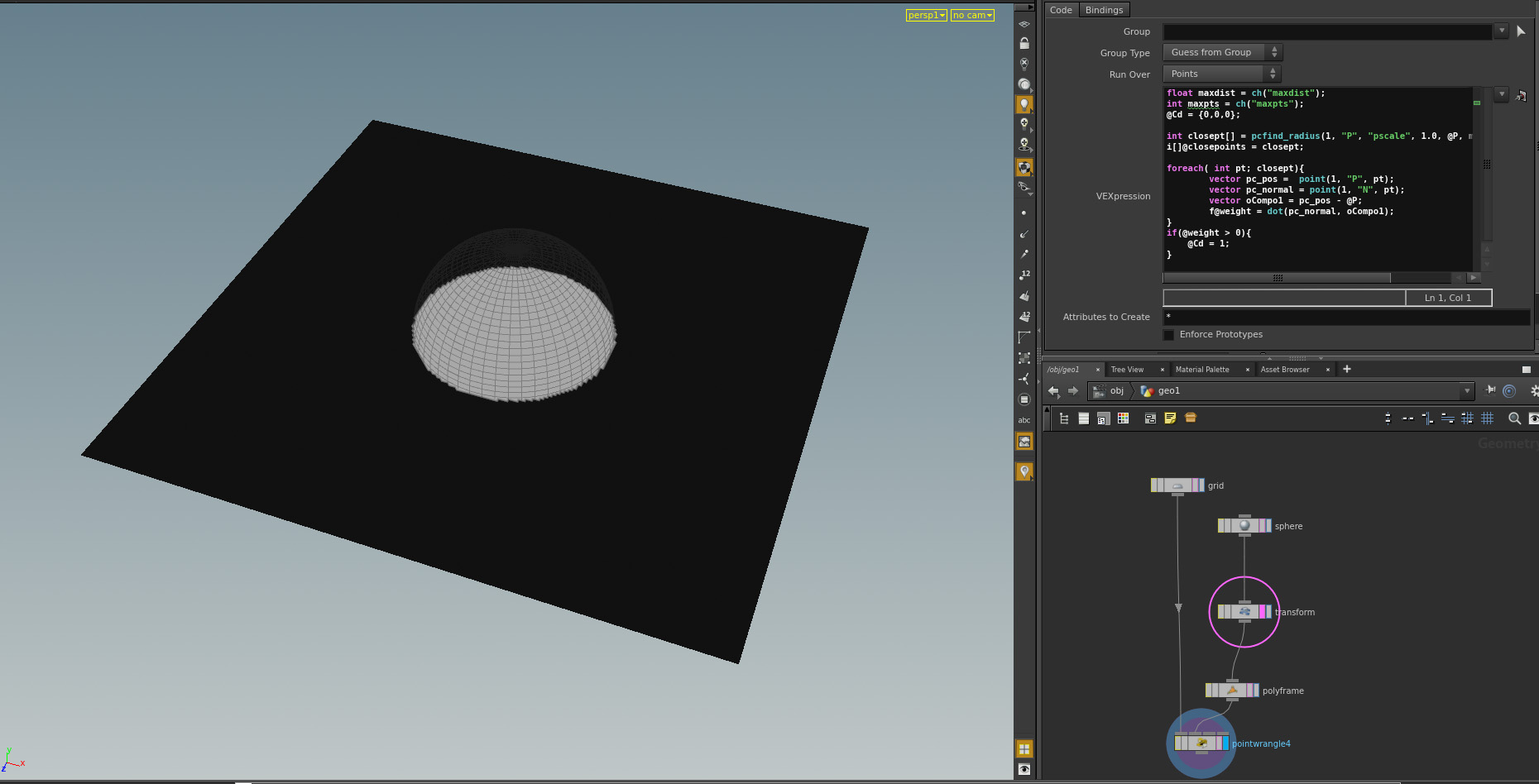# Point in Volume (Feat VEX)

Hey!

In my last post I showed a simple “Point in Volume” VOP. Inspired from the Side FX VEX Webinar I ported this logic to VEX with an attribute wrangle.```float maxdist = ch("maxdist");
int maxpts = ch("maxpts");
@Cd = {0,0,0};

int closept[] = pcfind_radius(1, "P", "pscale", 1.0, @P, maxdist, maxpts);
i[]@closepoints = closept;

foreach( int pt; closept){
vector pc_pos =  point(1, "P", pt);
vector pc_normal = point(1, "N", pt);
vector oCompo1 = pc_pos - @P;
f@weight = dot(pc_normal, oCompo1);
}
if(@weight > 0){
@Cd = 1;
}

```

Quite easy AND quite cool. Let’s explore VEX a little more  😉